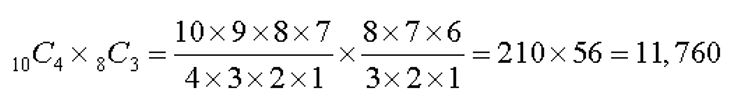# What do you mean by Choice Numbers ?

An online choice number definition

Choice numbers, more commonly called combination numbers, or simply combinations, are the number of ways that a number of things can be selected, chosen, or grouped. Combinations concern only the grouping of items and not the arrangement of those items. They typically evolve from the question how many combinations of “n” objects are possible using all “n” objects or “r” objects at a time?

To find the number of combinations of “n” dissimilar things taken “r” at a time, the formula is:which can be stated as “n” factorial divided by the product of “r” factorial times (n – r) factorial.

Example:

How many ways can a committee of four girls and three boys be selected from a class of ten girls and eight boys?

Results: